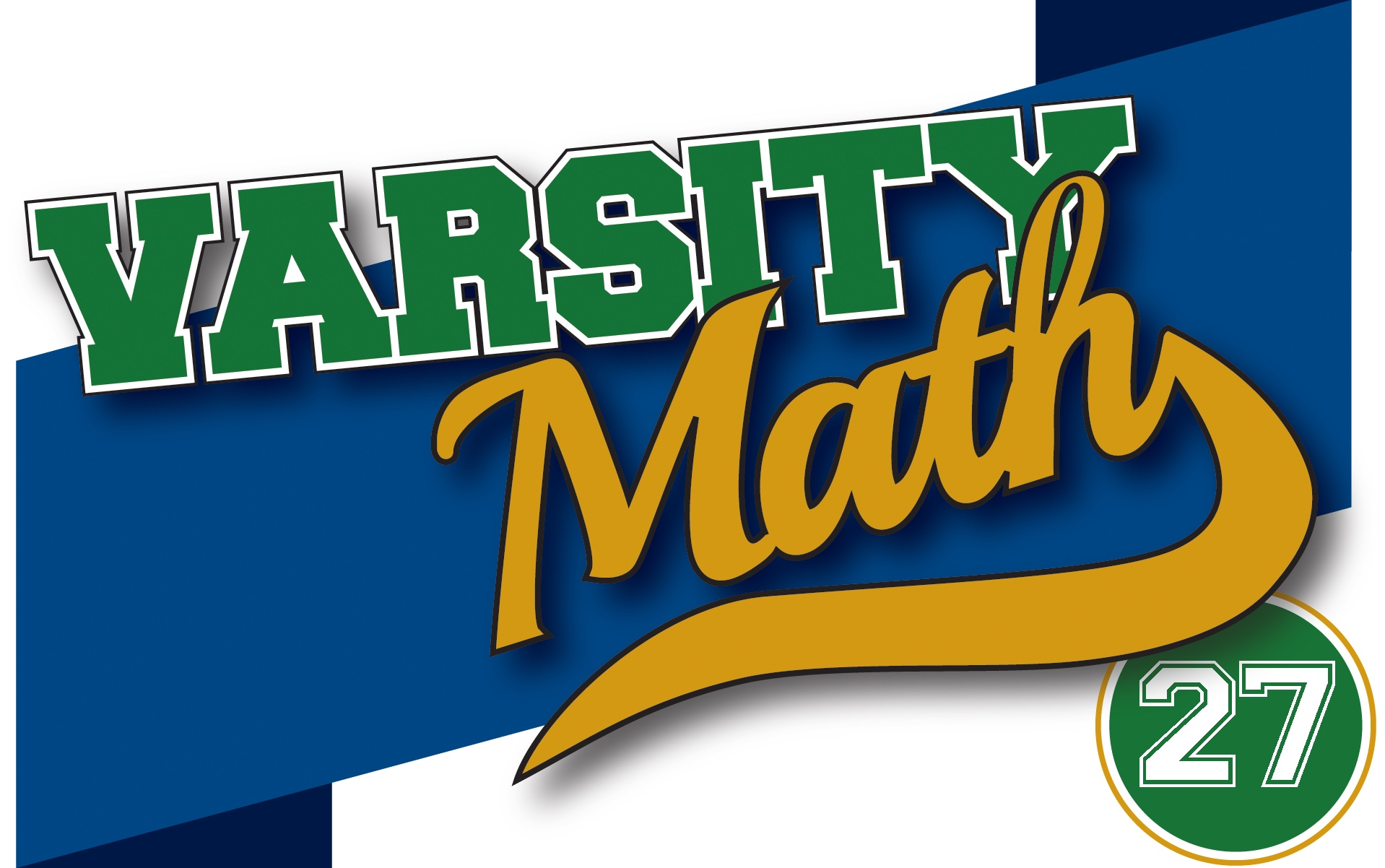This week, the team is helping out at a high school math tournament, and again a couple of problems caught their eye.

### Fancy Dice

A truncated tetrahedron (see the picturefor an example) or “trunctet” is constructed from four differently colored regular hexagons and four differently colored equilateral triangles.

How many distinct trunctets can be made from those same two sets of hexagons and triangles, if two trunctets are considered distinct if one cannot be rotated to exactly match the other?

### Gothic Arc

A circular arc has been drawn from point A to point C. Point B is the center of the circle of which AC is an arc. A different circular arc has been drawn from point B to point C. As it happens, point A is the center of the circle of which BC is an arc. There is a unique circle U which is tangent to both of these arcs AC and BC from the concave side of each arc, and to line segment AB. The two circular arcs each have length (along the arcs) equal to the square root of the numerator of the answer to last week’s Inscribed Square problem (when written in lowest terms).

What is the circumference of circle U?

## Solution to Week 26

HIJKMNOP.Because the smallest square S overlays one vertex of triangle abc, that is a right triangle, and hence by the Pythagorean theorem, we know that area A plus area B equals the area of C, the largest square. The desired area of the grey L-shaped region is clearly equal to area C minus area Z. But the problem gives us that B and Z are congruent, so area Z is equal to area B. Thus, the L-shaped region is area C minus area B, which in light of the above relation (subtracting area B from both sides of the equality) is just area A. Finally we observe that one edge of square A exactly matches three copies of the edge of smallest square S, which is given as a unit square. Hence the edge length of square A is three units, and so its area, which is equal to the area of the grey L, is 9 square units.